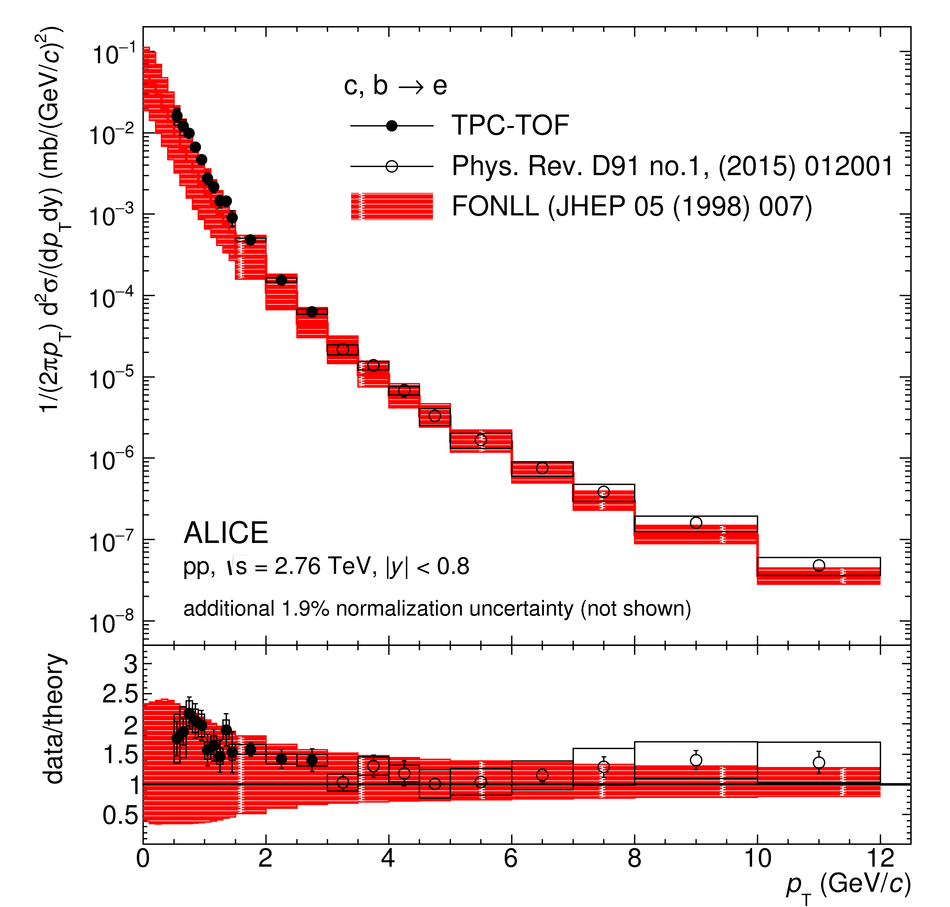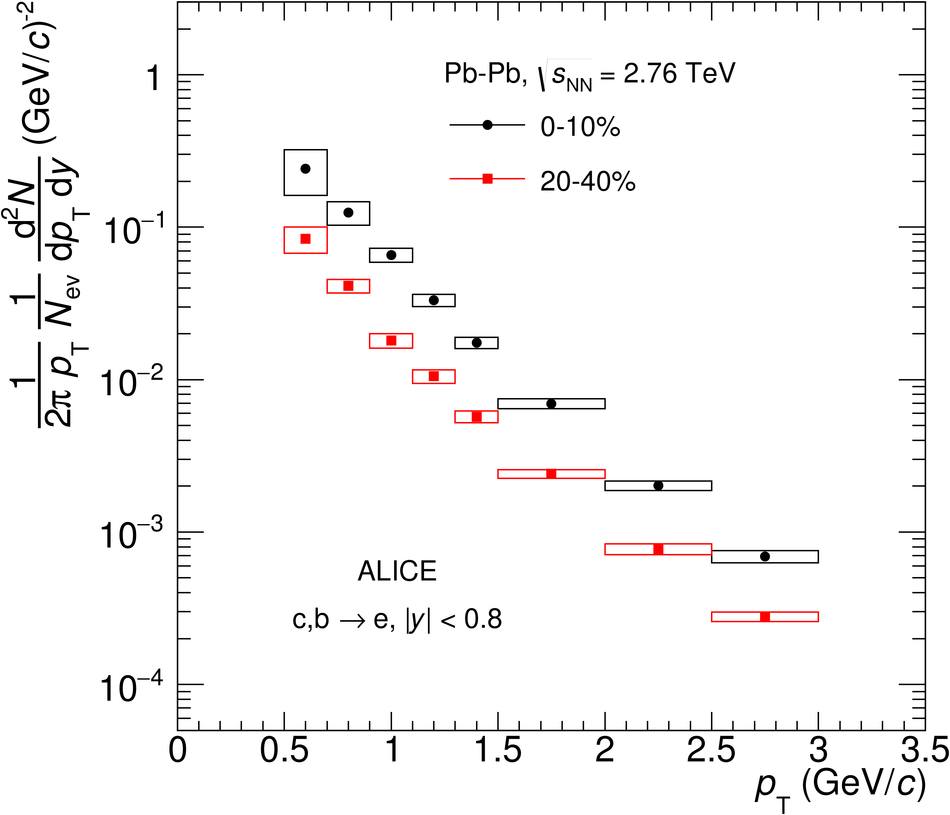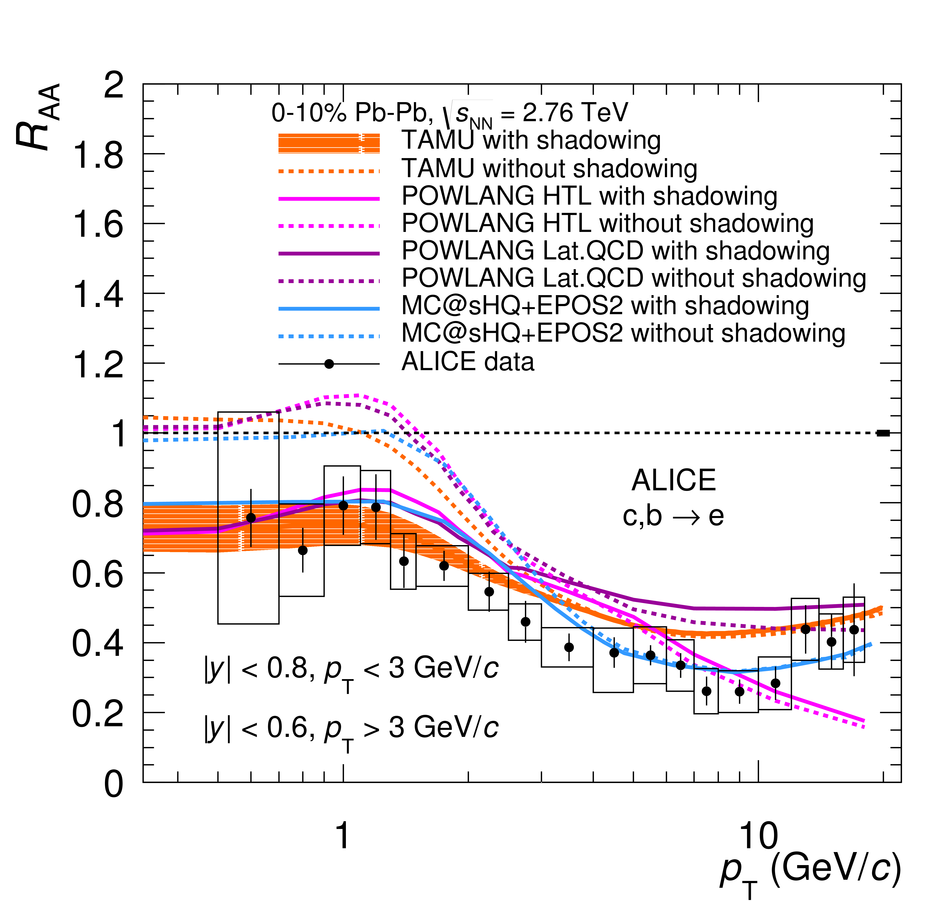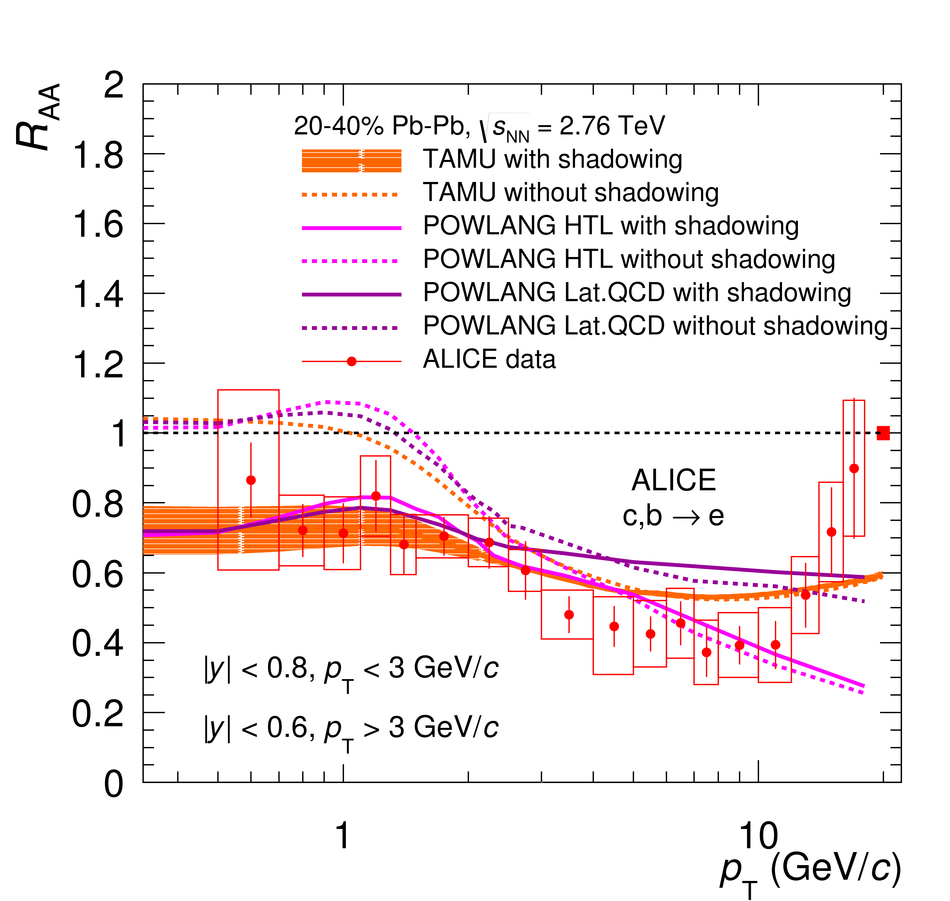Measurements of low-$p_{\rm T}$ electrons from semileptonic heavy-flavour hadron decays at mid-rapidity in pp and Pb-Pb collisions at $\mathbf{\sqrt{{\it s}_\mathrm{NN}}}$ = 2.76 TeV

Transverse-momentum ($p_{\rm T}$) differential yields of electrons from semileptonic heavy-flavour hadron decays have been measured in the most central (0-10%) and in semi-central (20-40%) Pb-Pb collisions at $\sqrt{s_{\rm NN}} = 2.76$ TeV. The corresponding production cross section in pp collisions has been measured at the same energy with substantially reduced systematic uncertainties with respect to previously published results. The modification of the yield in Pb-Pb collisions with respect to the expectation from an incoherent superposition of nucleon-nucleon collisions is quantified at mid-rapidity ($|y|$ $<~$ 0.8) in the $p_{\rm T}$ interval 0.5-3 GeV/$c$ via the nuclear modification factor, $R_{\rm AA}$. This paper extends the $p_{\rm T}$ reach of the $R_{\rm AA}$ measurement towards significantly lower values with respect to a previous publication. In Pb-Pb collisions the $p_{\rm T}$-differential measurements of yields at low $p_{\rm T}$ are essential to investigate the scaling of heavy-flavour production with the number of binary nucleon-nucleon collisions. Heavy-quark hadronization, a collective expansion and even initial-state effects, such as the nuclear modification of the Parton Distribution Functions, are also expected to have a significant effect on the measured distribution.

Figures

Figure 1

 The $p_{\rm T}$-differential invariant production cross section for electrons from semileptonicheavy-flavour hadron decays measured at mid-rapidity in pp collisions at $\sqrt{s} = 2.76$~TeV in comparison with FONLL pQCD calculations~ (upper panel), and the ratio of the data to the FONLL calculation (lower panel). Statistical and systematic uncertainties areshown as vertical bars and boxes, respectively.Figure 2

 The $p_{\rm T}$-differential invariant yields of electrons from semileptonic heavy-flavour hadron decays measured at mid-rapidity in 0--10\% and 20--40\% central Pb--Pb collisions at $\sqrt{s_{\rm NN}} = 2.76$~TeV. Statistical uncertainties are smaller than the symbol size and the systematic uncertainties are shown as boxes.Figure 3

 Nuclear modification factor $R_{\rm AA}$ for electrons from semileptonic heavy-flavour hadron decays at mid-rapidity as a function of $p_{\rm T}$ in 0--10\% (left panel) and 20--40\% central (right panel) Pb--Pb collisions at \energy~ = 2.76\,TeV. Error bars (open boxes) represent the statistical (systematic) uncertainties. The normalization uncertainties are represented by the boxes at $R_{\rm AA} = 1$. The previously published results from~ have been updated using a new glauber model calculation .Figure 4

 $R_{\rm AA}$ of electrons from semileptonic heavy-flavour hadron decays at mid-rapidity as a function of $p_{\rm T}$ in 0--10\% Pb--Pb collisions at \energy~ = 2.76\,TeV compared to model calculations~.Figure 5

 $R_{\rm AA}$ of electrons from semileptonic heavy-flavour hadron decays at mid-rapidity as a function of $p_{\rm T}$ in 0--10\% (left) and 20--40\% central (right) Pb--Pb collisions at \energy~ = 2.76\,TeV compared to model calculations~ with and without EPS09 shadowing parameterisations~.